CBSE Class 10 Sample Paper for 2024 Boards - Maths Standard

Class 10
Solutions of Sample Papers for Class 10 Boards

## ABCD is a parallelogram. Point P divides AB in the ratio 2:3 and point Q divides DC in the ratio 4:1. Prove that OC is half of OA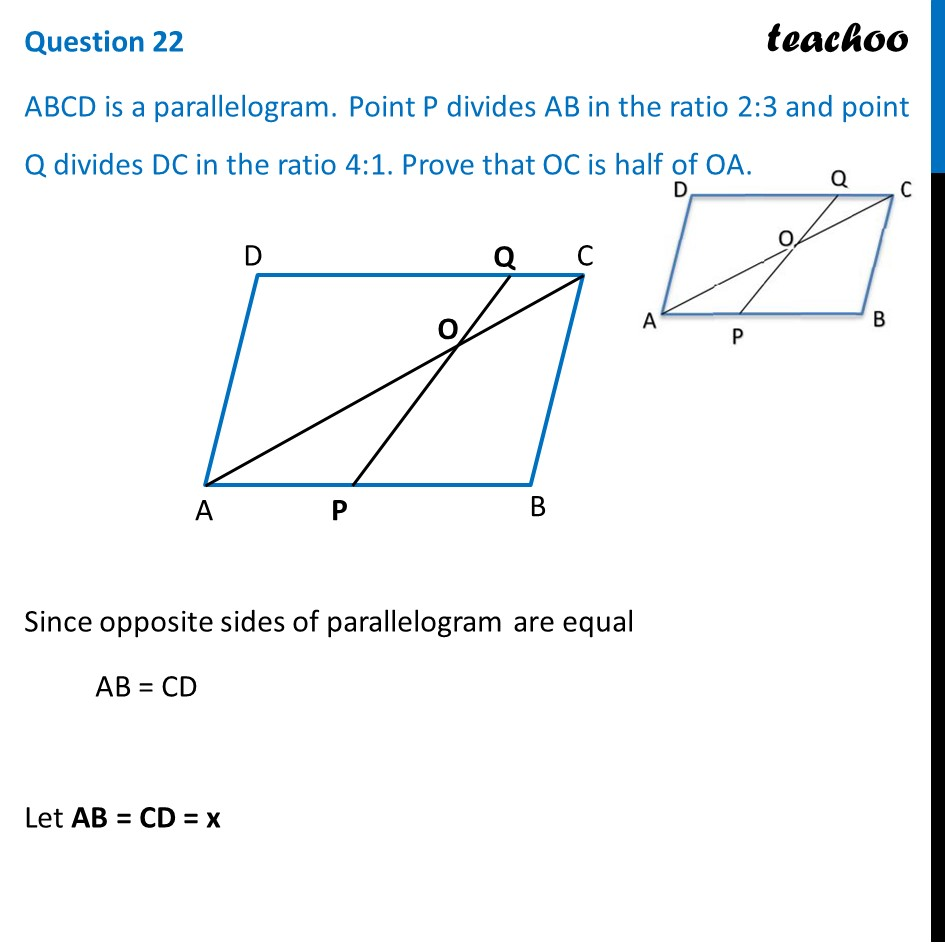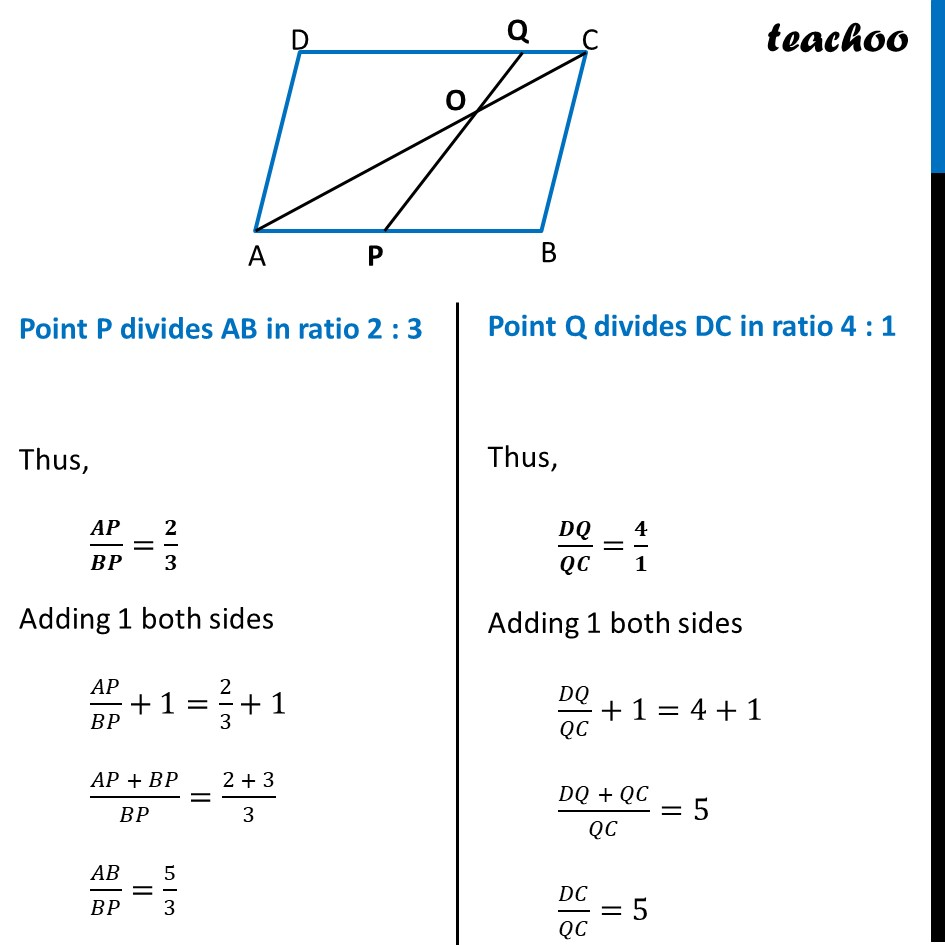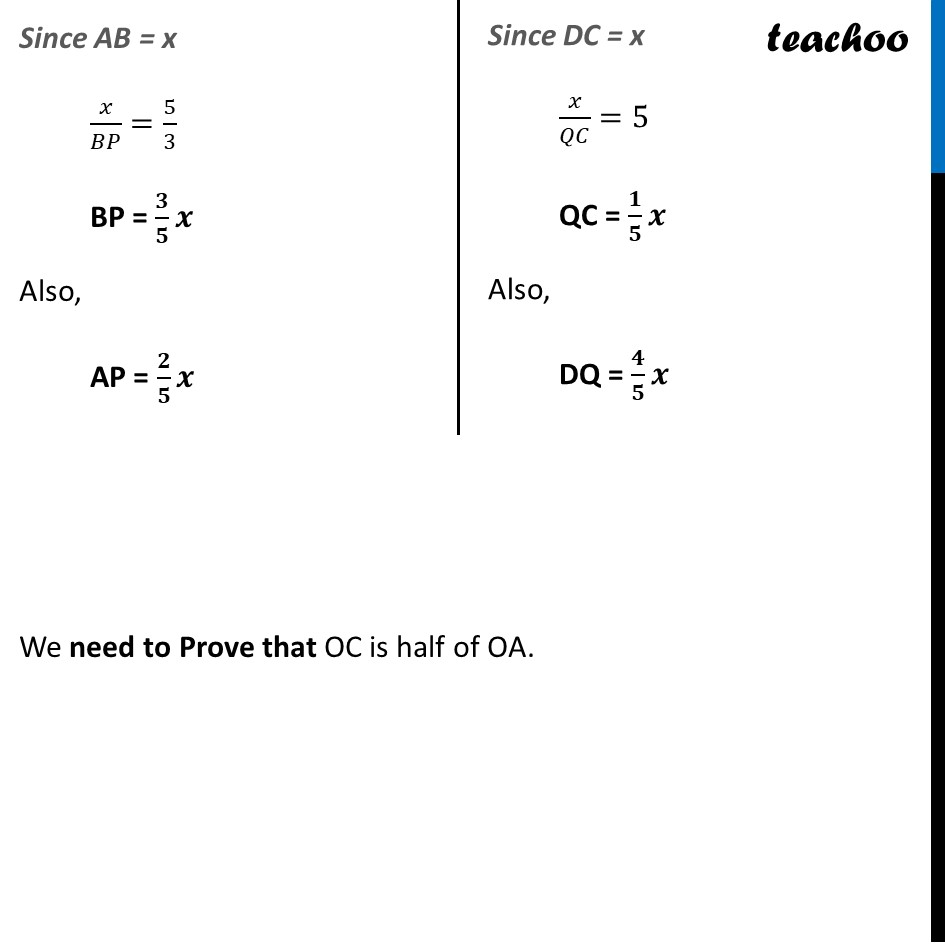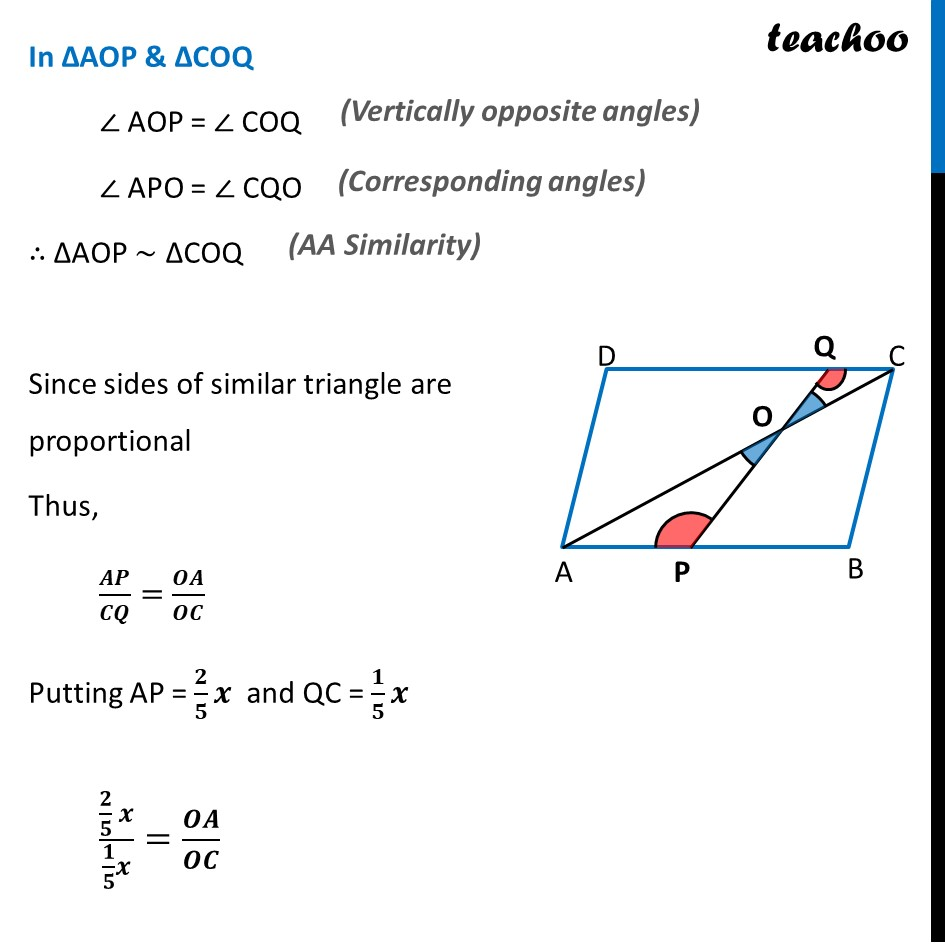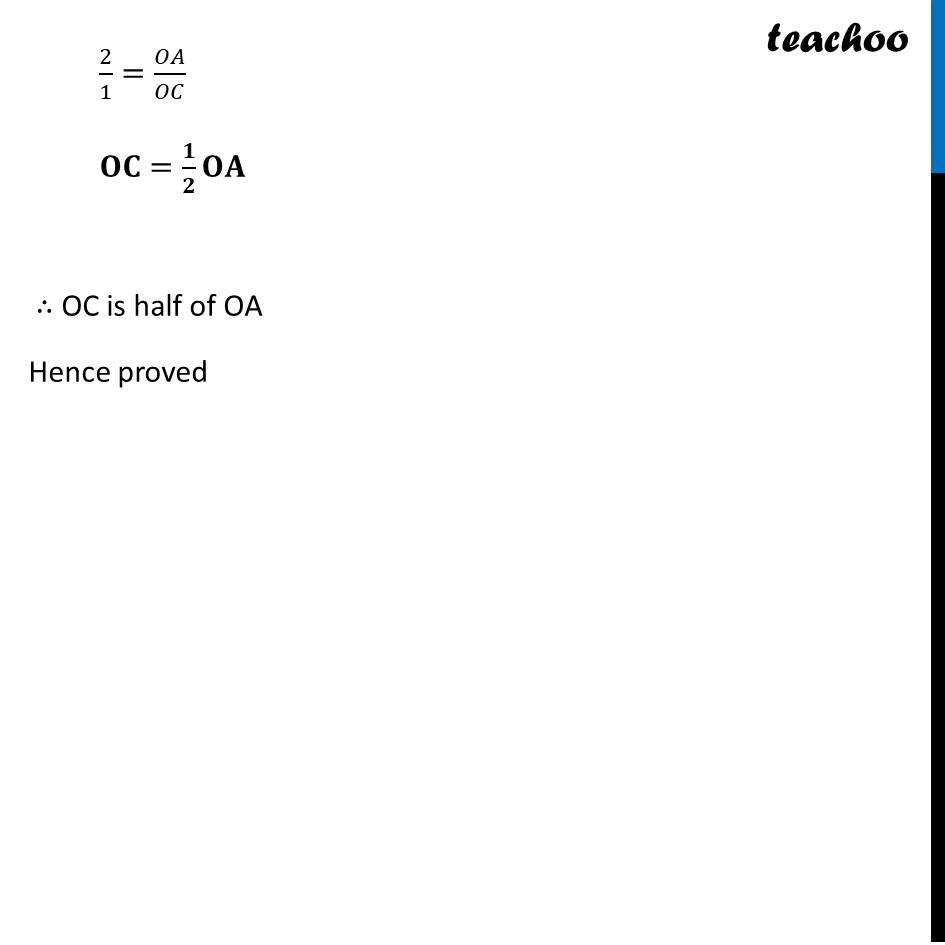Learn in your speed, with individual attention - Teachoo Maths 1-on-1 Class

### Transcript

Since opposite sides of parallelogram are equal AB = CD Let AB = CD = x Point P divides AB in ratio 2 : 3 Thus, 𝑨𝑷/𝑩𝑷=𝟐/𝟑 Adding 1 both sides 𝐴𝑃/𝐵𝑃+1=2/3+1 (𝐴𝑃 + 𝐵𝑃)/𝐵𝑃=(2 + 3)/3 𝐴𝐵/𝐵𝑃=5/3 Since AB = x 𝑥/𝐵𝑃=5/3 BP = 𝟑/𝟓 𝒙 Also, AP = 𝟐/𝟓 𝒙 Point P divides AB in ratio 2 : 3 Thus, 𝑨𝑷/𝑩𝑷=𝟐/𝟑 Adding 1 both sides 𝐴𝑃/𝐵𝑃+1=2/3+1 (𝐴𝑃 + 𝐵𝑃)/𝐵𝑃=(2 + 3)/3 𝐴𝐵/𝐵𝑃=5/3Point Q divides DC in ratio 4 : 1 Thus, 𝑫𝑸/𝑸𝑪=𝟒/𝟏 Adding 1 both sides 𝐷𝑄/𝑄𝐶+1=4+1 (𝐷𝑄 + 𝑄𝐶)/𝑄𝐶=5 𝐷𝐶/𝑄𝐶=5 In ΔAOP & ΔCOQ ∠ AOP = ∠ COQ ∠ APO = ∠ CQO ∴ ΔAOP ~ ΔCOQ Since sides of similar triangle are proportional Thus, 𝑨𝑷/𝑪𝑸=𝑶𝑨/𝑶𝑪 Putting AP = 𝟐/𝟓 𝒙 and QC = 𝟏/𝟓 𝒙 (𝟐/𝟓 𝒙)/(𝟏/𝟓 𝒙)=𝑶𝑨/𝑶𝑪 2/1=𝑂𝐴/𝑂𝐶 𝐎𝐂=𝟏/𝟐 𝐎𝐀 ∴ OC is half of OA Hence proved